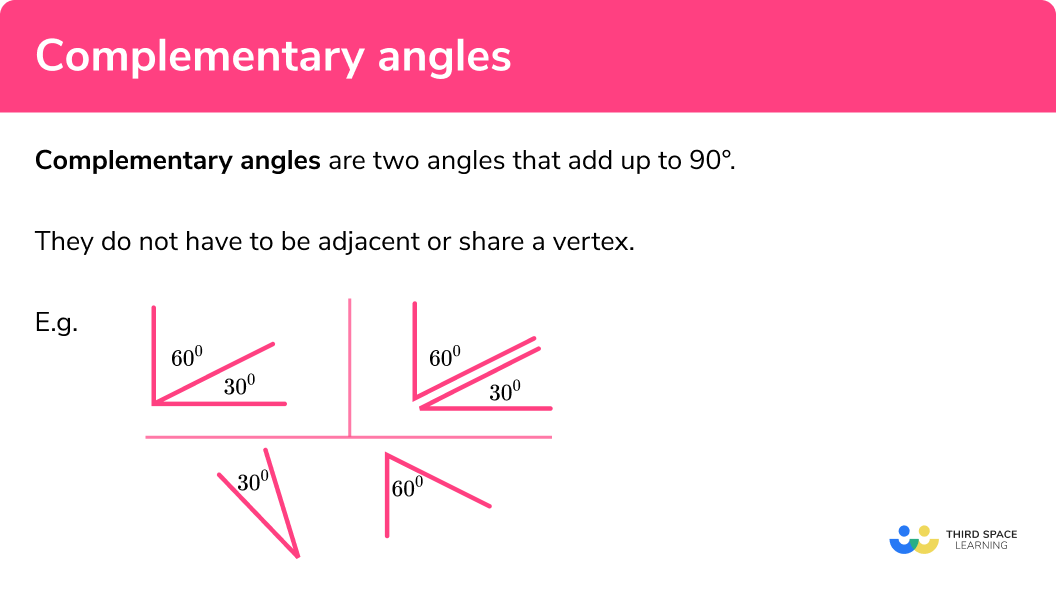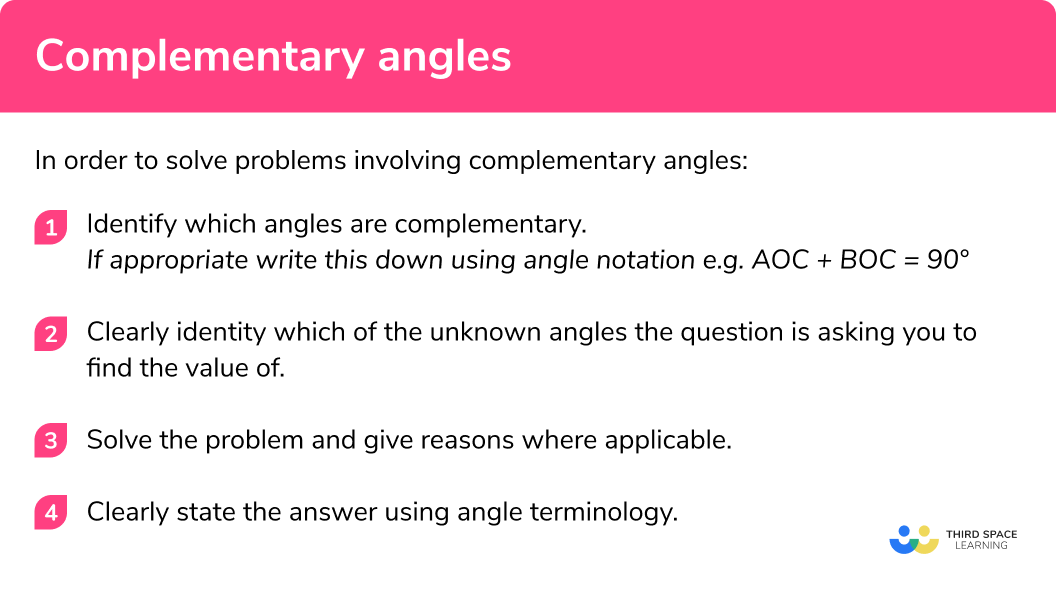# Complementary Angles

Here we will learn about complementary angles including how to find missing angles by applying knowledge of complementary angles to a context.

There are also angles worksheets based on Edexcel, AQA and OCR GCSE exam style questions, along with further guidance on where to go next if you’re still stuck.

## What are complementary angles?

Complementary angles are two angles that add up to 90 degrees. They do not have to be adjacent or share a vertex.

E.g.

When we add together complementary angles we get a right angle.

Before we start looking at specific examples it is important we are familiar with some key words, terminology, rules and symbols required for this topic:

• Angle: defined as the amount of turn around a common vertex.
• Vertex: the point created by two line segments (plural is vertices).

• How to label an angle:

We normally label angles in two main ways:

1. By giving the angle a ‘name’ which is normally a lowercase letter/symbol such as a, x or y or the greek letter (theta).

2By referring to the angle as the three letters that define the angle. The middle letter refers to the vertex at which the angle is e.g. see the diagram for the angle we call ABC:

• Angles on a straight line equal 180°:

Angles on one part of a straight line always add up to 180°.

However in the diagram below a and b do not equal 180° because they are not on one single part of a straight line, i.e. they do not share a vertex and are not adjacent to one another:

Angles around a point will always equal 360°. See the diagram for an example where angles a, b and c are equal to 360°:

• Vertically opposite angles:

Vertically opposite angles refer to angles that are opposite one another at a specific vertex and are created by two lines crossing. See below for an example:

Here the two angles labelled ‘a’ are equal to one another because they are ‘vertically opposite’ at the same vertex.

The same applies to angles labelled as ‘b’.

Note: Sometimes these are called vertical angles.

### What are complementary angles?## How to solve problems involving complementary angles

In order to solve problems involving complementary angles:

1. Identify which angles are complementary.
If appropriate write this down using angle notation e.g. AOC + BOC = 90°
2. Clearly identity which of the unknown angles the question is asking you to find the value of.
3. Solve the problem and give reasons where applicable.
4. Clearly state the answer using angle terminology.

### How to solve problems involving complementary angles## Complementary Angles examples

### Example 1: finding an angle which is complementary to another

Two angles ‘x and y‘ are complementary and one of them is 17°. What is the size of the other angle?

1. Identify which angles are complementary.

The two angles are complementary and therefore equal 90°

$x+y=90$

2Clearly identity which of the unknown angles the question is asking you to find the value of.

Find the angle that is not 17°.

3Solve the problem and give reasons where applicable.

\begin{aligned} x+y&=90 \\ x+17&=90 \\ x&=73 \end{aligned}

4Clearly state the answer using angle terminology.

The size of the other angle is 73°.

### Example 2: finding an angle which is complementary to another

Two angles are complementary. One is double the size of the other. What is the size of the smaller angle?

The two non-identified angles are complementary and therefore equal 90°.

You are being asked to find the smaller angle.

If you call the first angle ‘a‘ then the other angle must be 2a as ‘one is double the size of the other.’ Therefore a + 2a = 90.

We can now solve this equation:

\begin{aligned} a+2a&=90 \\ 3 a&=90 \hspace{3cm} \text{simplify the equation} \\ a&=30 \hspace{3cm} \text{divide each side of the equation by 3} \end{aligned}

The two angles are therefore of size 30° and 60°.

The smaller angle is 30°.

### Example 3: finding complementary angles from a diagram

ABC is a right-angled triangle. Which of the following pair of angles is complementary?

In this question you are not told which angles are complementary.

You are trying to find the angles that are complementary. Therefore we are looking for two angles that when added together equal 90°.

We know the sum of interior angles for a triangle is 180° and in a right angle triangle one of the angles is 90°. Consequently we can deduce that the other two angles must also be equal to 90° and therefore be complementary.

Angles ABC and BCA are complementary.

### Example 4: finding a given angle using complementary angles

Angles A and B are complementary to one another.

$A = 2x – 8$
$B = 5x – 7$

Find the size of angle A.

The two angles given as A and B are complementary and therefore equal 90°.

Therefore A + B = 90°.

Find the size of angle A.

We can create an equation from the information given:

\begin{aligned} A+B&=90 \\ 2 x-8+5 x-7&=90 \\ 7 x-15&=90 \\ 7 x&=105 \\ x&=15 \end{aligned}

Remember you need to find the value of angle A so we substitute the value x = 15 into the expression for angle A:

\begin{aligned} A=2x-8\\ A=2(15)-8\\ A=30-8\\ A=22 \end{aligned}

Angle A = 22°.

### Example 5: identifying complementary angles within a polygon

ABCD is a rectangle. Which of these is a pair of complementary angles: DAB and DCB or DAC and CAB?

In this question you are not told which angles are complementary.

You are trying to find the angles that are complementary and therefore you are looking for two angles that when added together equal 90°. Remember you are given a choice of DAB and DCB or DAC and CAB.

Below is the diagram (given in the question) where the two sets of angles have been labelled separately. This will help you spot which are complementary

DAB (top left angle) and DCB (bottom right angle)

The two angles labelled are both right angles as they are created by adjacent line segments of a rectangle. Therefore when added together they make 180 degrees, and are subsequently supplementary angles not complementary angles.

DAC (green angle) and CAB (blue angle)

The diagram shows the two angles make a right angle and are therefore complementary

Angle DAC and CAB are complementary.

### Example 6: complementary angles within a polygon

Can a parallelogram contain a pair of complementary angles? If so state their size.

In this question you are not told which angles are complementary.

You are being asked to find whether a parallelogram contains a pair of angles that when added together equal to 90°. A diagram is below:

The interior angles at vertex A and C are obtuse and therefore cannot be part of a complementary angle pair.

The interior angles at vertex D and B are equal to one another (congruent) and acute angles. Therefore to be complementary they would have to be 45° each.

A parallelogram can contain complementary angles.

The two acute angles in the parallelogram would be 45° each.

### Common misconceptions

• Incorrectly labelling angles which are complementary
• Assuming complementary angles must share a vertex
• Mixing up complementary angles and supplementary angles
• Misuse of the ‘straight line’ rule where angles do not share a vertex
• Finding the incorrect angle due to misunderstanding the terminology

Complementary angles is part of our series of lessons to support revision on angle rules. You may find it helpful to start with the main angle rules lesson for a summary of what to expect, or use the step by step guides below for further detail on individual topics. Other lessons in this series include:

### Practice complementary angles questions

1. Two angles ‘ x and y ‘ are complementary and one of them is 47^{\circ} . What is the size of the other angle?

x=33x=43x=133x=313If two angles are complementary, they add up to 90 , 43+47=90 .

2. Two angles ‘ x and y ‘ are complementary and one of them is 3^{\circ} . What is the size of the other angle?

x=3x=87x=93x=177If two angles are complementary, they add up to 90 , 3+87=90

3. Two angles are complementary. One is four times the size of the other. What is the size of the smaller angle?

20^{\circ}36^{\circ}18^{\circ}72^{\circ}The angles make up a 90 degree angle. There are four parts in one angle and one part in the other, so five parts in total. If we divide 90 by 5 we get 18 , so this is the size of the smaller angle.

4. Two angles are complementary. One is x – 16 and the other is 2x – 29 . Find the value of x .

x=16x=29x=45x=90The sum of the two angles must equal 90 , so the equation we must solve is 3x-45=90 . Using the standard methods for solving a linear equation gives the solution x=45 .

5. Two angles A and B are complementary. Find the difference between the two angles if: A=2x-33 and B= 5x-31

33^{\circ}22^{\circ}68^{\circ}79^{\circ}The sum of the two angles must equal 90 , so the equation we must solve is 7x-64=90 . Using the standard methods for solving a linear equation gives the solution x=22 so the two angles are 11^{\circ} and 79^{\circ}

6. Does a rectangle have any pairs of complementary angles?

YesNoSince each corner of a rectangle is a right angle, it is not possible for any two angles to add up to 90 .

### Complementary Angles GCSEquestions

1. Find the size of the angle marked a :(2 marks)

90-61

(1)

29^{\circ}

(1)

2. Are angles x and y complementary? Give reasons for your answer.(3 marks)

135 + 135 = 270

(1)

 360-270 = 90  - angles in a quadrilateral add to  360^{\circ}

(1)

Yes they are complementary as they add up to  90^{\circ}

(1)

3. Find the size of the larger angle:(4 marks)

3x + 5x + 2 = 90

(1)

8x + 2 = 90

(1)

\begin{aligned} 8x&=88\\ x&=11 \end{aligned}

(1)

5 \times 11 + 2 = 57

(1)

## Learning checklist

You have now learned how to:

• Use conventional terms and notation for angles
• Define angles that are complementary
• Apply the properties of complementary angles
• Apply angle facts and properties to solve problems

## Still stuck?

Prepare your KS4 students for maths GCSEs success with Third Space Learning. Weekly online one to one GCSE maths revision lessons delivered by expert maths tutors.

Find out more about our GCSE maths tuition programme.